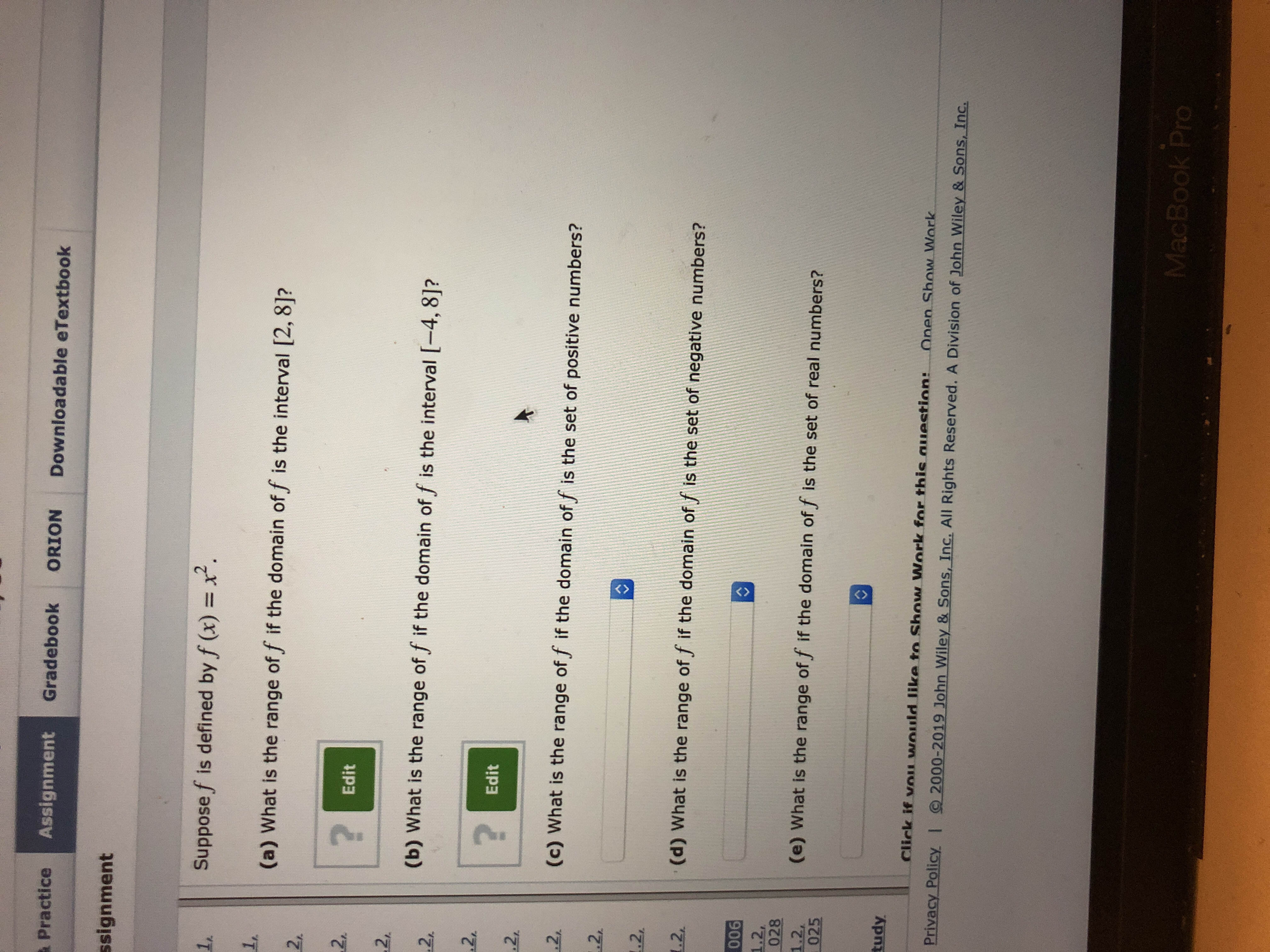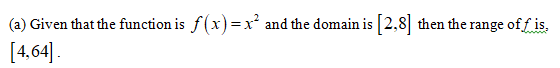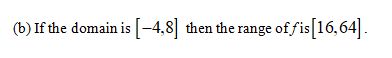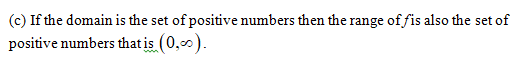GradebookORIONDownloadable eTextbookAssignmentPracticessignment2.Suppose f is defined by f (x)1,1,(a) What is the range of f if the domain of f is the interval [2, 8]?2r22 Edit2,(b) What is the range of f if the domain of f is the interval [-4, 8]?22.?Edit2(c) What is the range of f if the domain of f is the set of positive numbers?2,2.2(d) What is the range of f if the domain of f is the set of negative numbers?1.2,0061.2,0281.2,025(e) What is the range of f if the domain of f is the set of real numbers?tudyClick if vOu wOuld like to Show Work for this auuestion:Onen Show WorkPrivacy Policy I 2000-2019 John Wiley & Sons, Inc. All Rights Reserved. A Division of John Wiley & Sons, Inc.MacBook Pro

Question

How do I solve this?help_outlineImage TranscriptioncloseGradebook ORION Downloadable eTextbook Assignment Practice ssignment 2. Suppose f is defined by f (x) 1, 1, (a) What is the range of f if the domain of f is the interval [2, 8]? 2r 2 2 Edit 2, (b) What is the range of f if the domain of f is the interval [-4, 8]? 2 2. ? Edit 2 (c) What is the range of f if the domain of f is the set of positive numbers? 2, 2 .2 (d) What is the range of f if the domain of f is the set of negative numbers? 1.2, 006 1.2, 028 1.2, 025 (e) What is the range of f if the domain of f is the set of real numbers? tudy Click if vOu wOuld like to Show Work for this auuestion: Onen Show Work Privacy Policy I 2000-2019 John Wiley & Sons, Inc. All Rights Reserved. A Division of John Wiley & Sons, Inc. MacBook Pro fullscreen
Step 1Step 2Step 3...

Want to see the full answer?

See Solution

Want to see this answer and more?

Our solutions are written by experts, many with advanced degrees, and available 24/7

See Solution
Tagged in

Other